# Approximate Proximal Point Algorithms for Finding Zeroes of Maximal Monotone Operators in Hilbert Spaces

## Abstract

Letbe a real Hilbert space,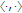a nonempty closed convex subset of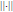, and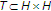a maximal monotone operator with. Letbe the metric projection of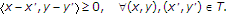onto. Suppose that, for any given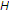,, and, there exists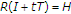satisfying the following set-valued mapping equation:for all, wherewithas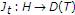and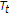is regarded as an error sequence such that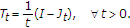. Let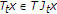be a real sequence such thatas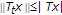and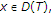. For any fixed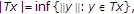, define a sequenceiteratively as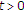for all. Thenconverges strongly to a point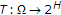as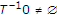, where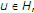.

## Author information

Authors

### Corresponding author

Correspondence to Haiyun Zhou.

## Rights and permissions

Reprints and Permissions

Cho, Y.J., Kang, S.M. & Zhou, H. Approximate Proximal Point Algorithms for Finding Zeroes of Maximal Monotone Operators in Hilbert Spaces. J Inequal Appl 2008, 598191 (2007). https://doi.org/10.1155/2008/598191

• Accepted:

• Published:

• DOI: https://doi.org/10.1155/2008/598191

### Keywords

• Hilbert Space
• Monotone Operator